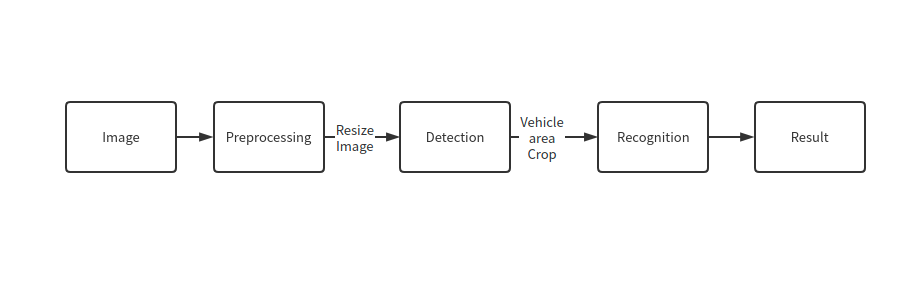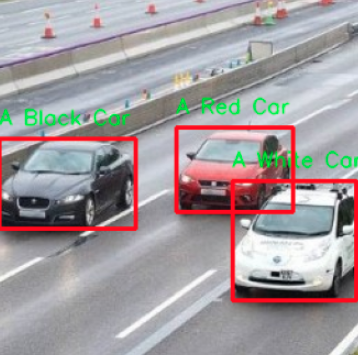# Vehicle Detection And Recognition with OpenVINO™¶

This tutorial is also available as a Jupyter notebook that can be cloned directly from GitHub. See the installation guide for instructions to run this tutorial locally on Windows, Linux or macOS.This tutorial demonstrates how to use two pre-trained models from Open Model Zoo: vehicle-detection-0200 for object detection and vehicle-attributes-recognition-barrier-0039 for image classification. Using these models, you will detect vehicles from raw images and recognize attributes of detected vehicles.As a result, you can get:result

# Imports¶

Import the required modules.

import os
import sys
from pathlib import Path
from typing import Tuple

import cv2
import numpy as np
import matplotlib.pyplot as plt
from openvino.runtime import Core

sys.path.append("../utils")
import notebook_utils as utils


Use omz_downloader - a command-line tool from the openvino-dev package. The omz_downloader tool automatically creates a directory structure and downloads the selected model. This step is skipped if the model is already downloaded. The selected model comes from the public directory, which means it must be converted into OpenVINO Intermediate Representation (OpenVINO IR).

Note: To change the model, replace the name of the model in the code below, for example to "vehicle-detection-0201" or "vehicle-detection-0202". Keep in mind that they support different image input sizes in detection. Also, you can change the recognition model to "vehicle-attributes-recognition-barrier-0042". They are trained from different deep learning frames. Therefore, if you want to change the precision, you need to modify the precision value in "FP32", "FP16", and "FP16-INT8". A different type has a different model size and a precision value.

# A directory where the model will be downloaded.
base_model_dir = "model"
# The name of the model from Open Model Zoo.
detection_model_name = "vehicle-detection-0200"
recognition_model_name = "vehicle-attributes-recognition-barrier-0039"
# Selected precision (FP32, FP16, FP16-INT8)
precision = "FP32"

# Check if the model exists.
detection_model_path = (
f"model/intel/{detection_model_name}/{precision}/{detection_model_name}.xml"
)
recognition_model_path = (
f"model/intel/{recognition_model_name}/{precision}/{recognition_model_name}.xml"
)

if not os.path.exists(detection_model_path):
f"--name {detection_model_name} " \
f"--precision {precision} " \
f"--output_dir {base_model_dir}"
! $download_command # Download the recognition model. if not os.path.exists(recognition_model_path): download_command = f"omz_downloader " \ f"--name {recognition_model_name} " \ f"--precision {precision} " \ f"--output_dir {base_model_dir}" !$download_command

################|| Downloading vehicle-detection-0200 ||################



This tutorial requires a detection model and a recognition model. After downloading the models, initialize OpenVINO Runtime, and use read_network to read network architecture and weights from *.xml and *.bin files. Then, compile it with compile_model() to the specified device.

# Initialize OpenVINO Runtime runtime.
ie_core = Core()

def model_init(model_path: str) -> Tuple:
"""
Read the network and weights from file, load the
model on the CPU and get input and output names of nodes

:param: model: model architecture path *.xml
:retuns:
input_key: Input node network
output_key: Output node network
exec_net: Encoder model network
net: Model network
"""

# Read the network and corresponding weights from a file.
# Compile the model for CPU (you can use GPU or MYRIAD as well).
compiled_model = ie_core.compile_model(model=model, device_name="CPU")
# Get input and output names of nodes.
input_keys = compiled_model.input(0)
output_keys = compiled_model.output(0)
return input_keys, output_keys, compiled_model


## Get attributes from model¶

Use input_keys.shape to get data shapes.

# de -> detection
# re -> recognition
# Detection model initialization.
input_key_de, output_keys_de, compiled_model_de = model_init(detection_model_path)
# Recognition model initialization.
input_key_re, output_keys_re, compiled_model_re = model_init(recognition_model_path)

# Get input size - Detection.
height_de, width_de = list(input_key_de.shape)[2:]
# Get input size - Recognition.
height_re, width_re = list(input_key_re.shape)[2:]


## Helper function¶

The plt_show() function is used to show image.

def plt_show(raw_image):
"""
Use matplot to show image inline
raw_image: input image

:param: raw_image:image array
"""
plt.figure(figsize=(10, 6))
plt.axis("off")
plt.imshow(raw_image)


## Read and display a test image¶

The input shape of detection model is [1, 3, 256, 256]. Therefore, you need to resize the image to 256 x 256, and expand the batch channel with expand_dims function.

# Load an image.
url = "https://storage.openvinotoolkit.org/data/test_data/images/person-bicycle-car-detection.bmp"
filename = "cars.jpg"
directory = "data"
url, filename=filename, directory=directory, show_progress=False, silent=True,timeout=30
)
assert Path(image_file).exists()

# Read the image.
# Resize it to [3, 256, 256].
resized_image_de = cv2.resize(image_de, (width_de, height_de))
# Expand the batch channel to [1, 3, 256, 256].
input_image_de = np.expand_dims(resized_image_de.transpose(2, 0, 1), 0)
# Show the image.
plt_show(cv2.cvtColor(image_de, cv2.COLOR_BGR2RGB))# Use the Detection Model to Detect Vehicles¶pipline

As shown in the flowchart, images of individual vehicles are sent to the recognition model. First, use infer function to get the result.

The detection model output has the format [image_id, label, conf, x_min, y_min, x_max, y_max], where:

• image_id - ID of the image in the batch

• label - predicted class ID (0 - vehicle)

• conf - confidence for the predicted class

• (x_min, y_min) - coordinates of the top left bounding box corner

• (x_max, y_max) - coordinates of the bottom right bounding box corner

Delete unused dims and filter out results that are not used.

# Run inference.
boxes = compiled_model_de([input_image_de])[output_keys_de]
# Delete the dim of 0, 1.
boxes = np.squeeze(boxes, (0, 1))
# Remove zero only boxes.
boxes = boxes[~np.all(boxes == 0, axis=1)]


## Detection Processing¶

With the function below, you change the ratio to the real position in the image and filter out low-confidence results.

def crop_images(bgr_image, resized_image, boxes, threshold=0.6) -> np.ndarray:
"""
Use bounding boxes from detection model to find the absolute car position

:param: bgr_image: raw image
:param: resized_image: resized image
:param: boxes: detection model returns rectangle position
:param: threshold: confidence threshold
:returns: car_position: car's absolute position
"""
# Fetch image shapes to calculate ratio
(real_y, real_x), (resized_y, resized_x) = bgr_image.shape[:2], resized_image.shape[:2]
ratio_x, ratio_y = real_x / resized_x, real_y / resized_y

# Find the boxes ratio
boxes = boxes[:, 2:]
# Store the vehicle's position
car_position = []
# Iterate through non-zero boxes
for box in boxes:
# Pick confidence factor from last place in array
conf = box
if conf > threshold:
# Convert float to int and multiply corner position of each box by x and y ratio
# In case that bounding box is found at the top of the image,
# we position upper box bar little bit lower to make it visible on image
(x_min, y_min, x_max, y_max) = [
int(max(corner_position * ratio_y * resized_y, 10)) if idx % 2
else int(corner_position * ratio_x * resized_x)
for idx, corner_position in enumerate(box[1:])
]

car_position.append([x_min, y_min, x_max, y_max])

return car_position

# Find the position of a car.
car_position = crop_images(image_de, resized_image_de, boxes)


## Recognize vehicle attributes¶

Select one of the detected boxes. Then, crop to an area containing a vehicle to test with the recognition model. Again, you need to resize the input image and run inference.

# Select a vehicle to recognize.
pos = car_position
# Crop the image with [y_min:y_max, x_min:x_max].
test_car = image_de[pos:pos, pos:pos]
# Resize the image to input_size.
resized_image_re = cv2.resize(test_car, (width_re, height_re))
input_image_re = np.expand_dims(resized_image_re.transpose(2, 0, 1), 0)
plt_show(cv2.cvtColor(resized_image_re, cv2.COLOR_BGR2RGB))### Recognition processing¶

The result contains colors of the vehicles (white, gray, yellow, red, green, blue, black) and types of vehicles (car, bus, truck, van). Next, you need to calculate the probability of each attribute. Then, you determine the maximum probability as the result.

def vehicle_recognition(compiled_model_re, input_size, raw_image):
"""
Vehicle attributes recognition, input a single vehicle, return attributes
:param: compiled_model_re: recognition net
:param: input_size: recognition input size
:param: raw_image: single vehicle image
:returns: attr_color: predicted color
attr_type: predicted type
"""
# An attribute of a vehicle.
colors = ['White', 'Gray', 'Yellow', 'Red', 'Green', 'Blue', 'Black']
types = ['Car', 'Bus', 'Truck', 'Van']

# Resize the image to input size.
resized_image_re = cv2.resize(raw_image, input_size)
input_image_re = np.expand_dims(resized_image_re.transpose(2, 0, 1), 0)

# Run inference.
# Predict result.
predict_colors = compiled_model_re([input_image_re])[compiled_model_re.output(1)]
# Delete the dim of 2, 3.
predict_colors = np.squeeze(predict_colors, (2, 3))
predict_types = compiled_model_re([input_image_re])[compiled_model_re.output(0)]
predict_types = np.squeeze(predict_types, (2, 3))

attr_color, attr_type = (colors[np.argmax(predict_colors)],
types[np.argmax(predict_types)])
return attr_color, attr_type

print(f"Attributes:{vehicle_recognition(compiled_model_re, (72, 72), test_car)}")

Attributes:('Gray', 'Car')


## Combine two models¶

Congratulations! You successfully used a detection model to crop an image with a vehicle and recognize the attributes of a vehicle.

def convert_result_to_image(compiled_model_re, bgr_image, resized_image, boxes, threshold=0.6):
"""
Use Detection model boxes to draw rectangles and plot the result

:param: compiled_model_re: recognition net
:param: input_key_re: recognition input key
:param: bgr_image: raw image
:param: resized_image: resized image
:param: boxes: detection model returns rectangle position
:param: threshold: confidence threshold
:returns: rgb_image: processed image
"""
# Define colors for boxes and descriptions.
colors = {"red": (255, 0, 0), "green": (0, 255, 0)}

# Convert the base image from BGR to RGB format.
rgb_image = cv2.cvtColor(bgr_image, cv2.COLOR_BGR2RGB)

# Find positions of cars.
car_position = crop_images(image_de, resized_image, boxes)

for x_min, y_min, x_max, y_max in car_position:
# Run vehicle recognition inference.
attr_color, attr_type = vehicle_recognition(compiled_model_re, (72, 72),
image_de[y_min:y_max, x_min:x_max])

# Close the window with a vehicle.
plt.close()

# Draw a bounding box based on position.
# Parameters in the rectangle function are: image, start_point, end_point, color, thickness.
rgb_image = cv2.rectangle(rgb_image, (x_min, y_min), (x_max, y_max), colors["red"], 2)

# Print the attributes of a vehicle.
# Parameters in the putText function are: img, text, org, fontFace, fontScale, color, thickness, lineType.
rgb_image = cv2.putText(
rgb_image,
f"{attr_color} {attr_type}",
(x_min, y_min - 10),
cv2.FONT_HERSHEY_SIMPLEX,
2,
colors["green"],
10,
cv2.LINE_AA
)

return rgb_image

plt_show(convert_result_to_image(compiled_model_re, image_de, resized_image_de, boxes))